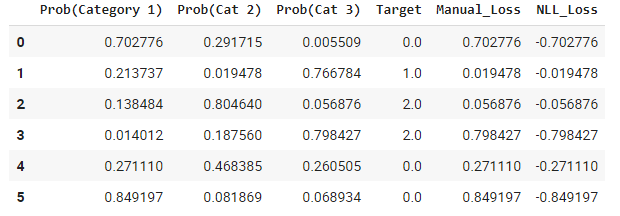# 05_pet_breeds: Understanding nll_loss() function

In the chapter, it is showed that for Multi-Category Classification we have to use `nll_loss()` function instead of `torch.where()`, that was used in 04_mnist_basics.
In the chapter it is also shown how to calculate `nll_loss()` manually.

For a multi-category classification we have the loss as shown:Code for above table
``````torch.random.manual_seed(42)
acts = torch.randn((6,3))*2
sm_acts = torch.softmax(acts,dim = 1)
targ = torch.tensor([0,1,2,2,0,0])
idx = range(6)

# loss calculate manually
loss = sm_acts[idx, targ]

# loss calculate using nll_loss()
nll_loss = torch.nn.functional.nll_loss(sm_acts, targ, reduction='none')

# Combine all to form a table
combine = torch.cat((sm_acts, targ.view(-1,1), loss.view(-1,1), nll_loss.view(-1,1)),1)
pd.DataFrame(np.array(combine), columns = ['Category 1', 'Category 2', 'Category 3', 'Target', 'Loss', 'NLL_Loss'])
``````

I have 3 questions:

1. Which of the loss (manually calculated or `nll_loss()`) is the actual loss here?
2. If manual_loss() is the actual loss, then in the image above, 1st row shows that Category 1 is the most probable, then why is loss also equal to the `probability`. Shouldn’t it be
`(1-probability)` just like calculated in 04_mnist_basics?
3. If `nll_loss()` is the actual loss, how should I interpret it? More negative value implies higher loss or less negative value implies higher loss?

My understanding is as follows:

1. The probabilities you are reporting are the output of softmax. Remember that you have to take the log of these values to get log_softmax first and then apply nll_loss to them.
2. nll_loss(log_softmax) will be the column you want to focus on as the final loss column/list
3. the final loss is actually the mean of the final loss column/list

In your code, you are missing the following:

log_sm_acts = torch.log(sm_acts)
nll_loss = -log_sm_acts[idx,targ]
OR
nll_loss = F.nll_loss(log_sm_acts, targ, reduction=‘none’)
OR nll_loss = torch.nn.functional.nll_loss(log_sm_acts, targ, reduction=‘none’)

In your case, the loss is equal to the probability as you have not taken the log of the softmax output. Also, log will spread the numbers from -inf to +inf as opposed to from 0 to 1 (which is what softmax does). From an interpretation perspective, numbers closer to 0 in the softmax output will be smaller than the numbers closer to 1 in the softmax output.

Hope that helps.

1 Like

First of all, this is not the loss. This are the activations of the correct classes. This are what our model predicted for the ground truth classes.

So the question then becomes, how do we calculate the loss from this values? Cross entropy requires us to take the log of this values and return the negative log likelihoods.

So the first step would be to calculate the log of these values (from softmax). When you calculate the log of a small number, e.g `log(0.702766)` from the prediction of the ground truth in the first row of your example, we get ` −0.153183078`. We get a negative number, which is not so useful in interpreting the loss, that’s why, after applying log to the softmax values, we go ahead and get the `negative_log_likelihoods` or `nll`. Which in this case would return `0.153183078`. The reason we only do this to the prediction of the ground-truth class is, values normalized by softmax always add up to one. Therefore, making the loss of the ground-truth class as small as possible means the other ones are increasing, therefore the model is getting better.

None, or at least not yet. If you look at your `Manual Loss` column, the values are exactly values from the correct class. And values from `NLL_Loss` column are the negated values of the `Manual Loss`. Apply log first then get `nll` loss. Then use `nn.CrossEntropy(reduction='none)` from PyTorch and compare the values (They should be equal)
Since `nll` is applied after taking the log, the values returned should be positive and you would interpret them normally.